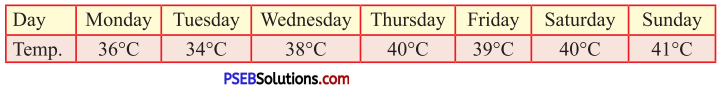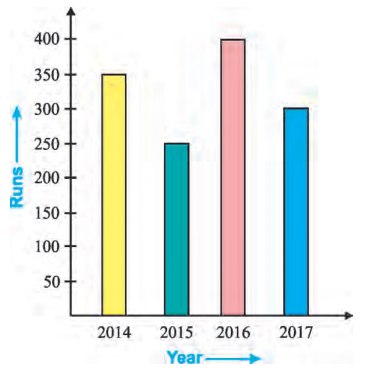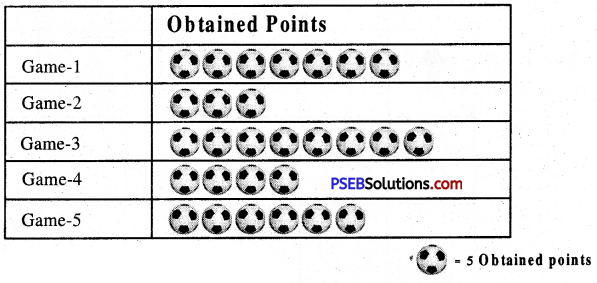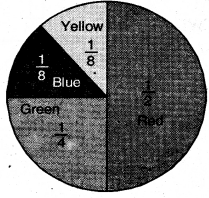# PSEB 5th Class Maths MCQ Chapter 10 Data Handling

Punjab State Board PSEB 5th Class Maths Book Solutions Chapter 10 Data Handling MCQ Questions and Answers.

## PSEB 5th Class Maths Chapter 10 Data Handling MCQ Questions

Multiple Choice Questions

Tick (✓) the correct answer :

1. The table shows the data of temperature of a city for a week :Question 1.
Which day is the hottest?
(a) Tuesday
(b) Thursday
(c) Sunday
(d) Monday.
(c) Sunday

Question 2.
Which day is the coldest?
(a) Wednesday
(b) Tuesday
(c) Saturday
(d) Friday.
(b) TuedayQuestion 3.
What is the difference between the highest and the lowest temperature?
(a) 6°C
(b) 8°C
(c) 5°C
(d) 7°C.
(d) 7°C

Question 4.
Which two days are equally hot?
(a) Thursday, Saturday
(b) Sunday, Monday
(c) Tuesday, Wednesday
(d) Thursday, Friday.
(a) Thursday, Saturday

2. The runs scored by a cricketer in four consecutive years are as follows :Question 1.
How many runs were scored by the player in 2016?
(a) 300
(b) 400
(c) 350
(d) 200.
(b) 400Question 2.
In which year, the player scored the least runs?
(a) 2017
(b) 2016
(c) 2015
(d) 2014.
(c) 2015

Question 3.
What is the difference of highest and lowest runs scored by a player?
(a) 150
(b) 50
(c) 200
(d) 100.
(a) 150

Question 4.
How many total runs he scored in four consecutive years?
(a) 1100
(b) 1000
(c) 1300
(d) 1200.
(c) 1300Question 3.
See the diagram. How many points were obtained for game 3.(a) 35
(b) 40
(c) 30
(d) 25.
Ans:
(b)

4. The given pie chart provide the information of colour liked by students of class five. Observe the pie chart carefully and answer the questions.Question 1.
How many students (in fractions) of class five like green colour?
Solution:
Number of students who like green colour = $$\frac{1}{4}$$ × 40 = 10

Question 2.
Which colour is liked by the students the most?
Solution:
The colour which is liked the most = RedQuestion 3.
If there are 40 students in the class, then how many of them like green colour?
Solution:
The number of students who like green colour = 40 × $$\frac{1}{4}$$ = 10